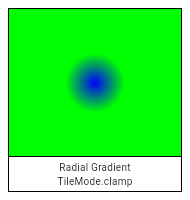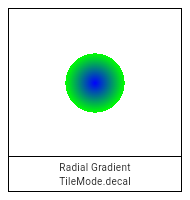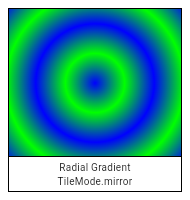1. Offset center,
3. colors,
4. [List<double>? colorStops,
5. TileMode tileMode = TileMode.clamp,
6. matrix4,
7. focal,
)

Creates a radial gradient centered at `center` that ends at `radius` distance from the center.

If `colorStops` is provided, `colorStops[i]` is a number from 0.0 to 1.0 that specifies where `color[i]` begins in the gradient. If `colorStops` is not provided, then only two stops, at 0.0 and 1.0, are implied (and `color` must therefore only have two entries).

The behavior before and after the radius is described by the `tileMode` argument. For details, see the TileMode enum.If `center`, `radius`, `colors`, or `tileMode` are null, or if `colors` or `colorStops` contain null values, this constructor will throw a NoSuchMethodError.

If `matrix4` is provided, the gradient fill will be transformed by the specified 4x4 matrix relative to the local coordinate system. `matrix4` must be a column-major matrix packed into a list of 16 values.

If `focal` is provided and not equal to `center` and `focalRadius` is provided and not equal to 0.0, the generated shader will be a two point conical radial gradient, with `focal` being the center of the focal circle and `focalRadius` being the radius of that circle. If `focal` is provided and not equal to `center`, at least one of the two offsets must not be equal to Offset.zero.

## Implementation

``````Gradient.radial(
Offset center,
List<Color> colors, [
List<double>? colorStops,
TileMode tileMode = TileMode.clamp,
Float64List? matrix4,
Offset? focal,
]) : assert(_offsetIsValid(center)),
assert(matrix4 == null || _matrix4IsValid(matrix4)),
super._() {
_validateColorStops(colors, colorStops);
final Int32List colorsBuffer = _encodeColorList(colors);
final Float32List? colorStopsBuffer = colorStops == null ? null : Float32List.fromList(colorStops);

// If focal is null or focal radius is null, this should be treated as a regular radial gradient
// If focal == center and the focal radius is 0.0, it's still a regular radial gradient
if (focal == null || (focal == center && focalRadius == 0.0)) {
_constructor();